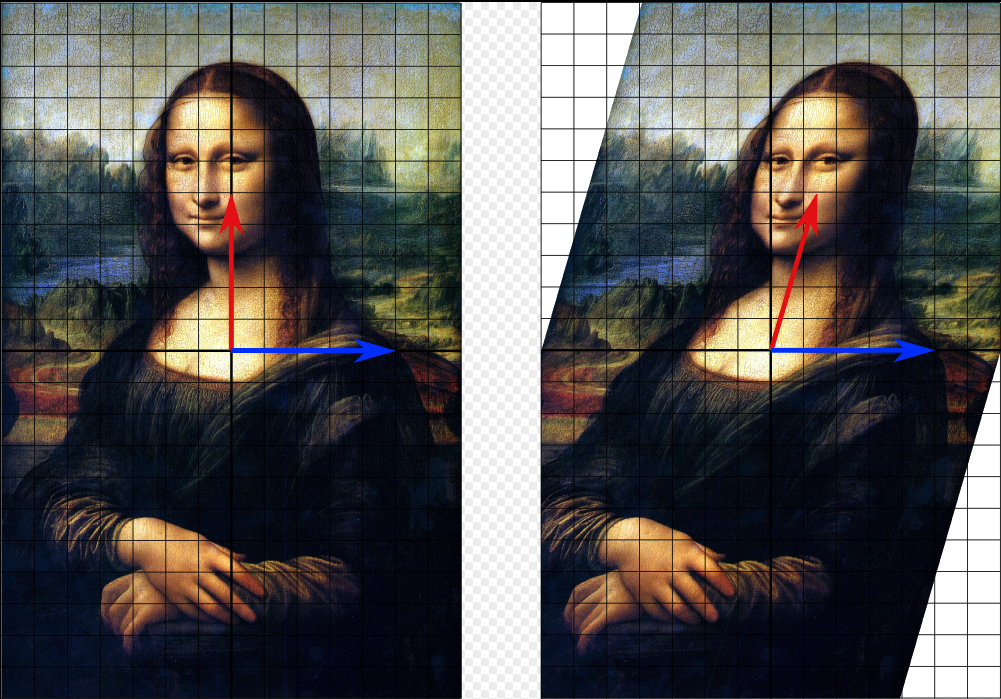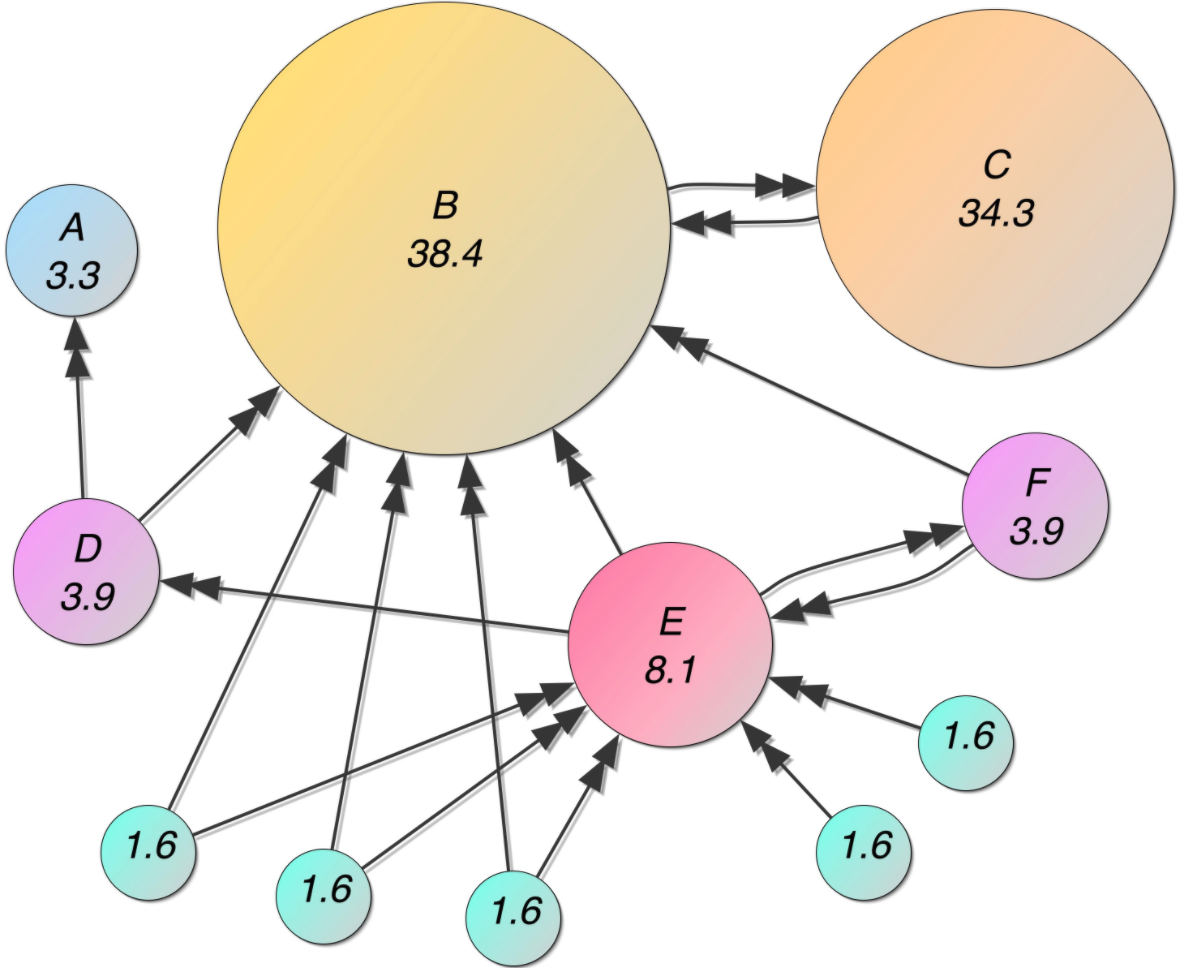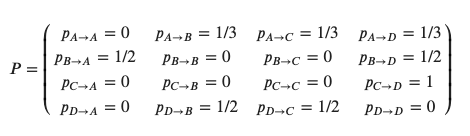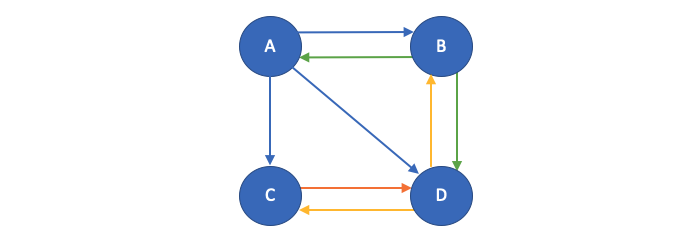### Eigenvalue, Eigenvector, Eigenspace and Implementation of Google's PageRank Algorithm

Posted July 3, 2021 by Gowri Shankar  ‐  8 min read

Feature extraction techniques like Principal Component Analysis use eigenvalues and vectors for dimensionality reduction in a machine learning model by density estimation process through eigentheory. Eigenvalues depicts the variance of distribution of data in certain direction, the vector having the highest eigenvalue is the principal component of the feature set. In simple terms, eigenvalues helps us to find patterns inside a noisy data. By the way, Eigen is a German word and it means Particular or Proper - When it combined with value, it means - the proper value.

Eigentheory has various applications from designing bridges to car stereo systems and oil exploration to face recognition. In this post, we shall explore the math behind eigenvalues and eigenvectors and understand their significance in the context of Principal Component Analysis in the first part. Subsequently, we shall implement Google’s PageRank algorithm under a controlled setup. This is the 6th post in the Math for ML series. Please refer here for other posts,### Objectives

• Introduce dimensionality reduction as a concept for feature extraction
• Explore eigentheory under the context of PCA
• Understand transformations and basis vectors along with NULL space
• Implement Page Rank algorithm under a controlled setup

## Introduction

Understanding Principal Component Analysis ensures understanding of significant number of fascinating topics of linear algebra in detail. The process of identifying a Principal components involves

• Matrix Multiplication, Transpose and Inverse
• Matrix Decomposition
• Eigen Values, Vectors and Spaces

Further, it includes everyday statistics like

• Standard Deviation
• Variance
• Covariance
• Independence
• Linear Regression etc

We seek PCA when we have large number of feature vectors in our dataset and there is a need to eliminate unwanted feature vectors to train an impactful model. This process of extracting(not eliminating) features from a large feature vector is called as dimensionality reduction, imagine each feature encompass a dimension space. For example,

• A point, is the hyperplane on a 1 dimensional space
• Similarly, A line is the hyperplane on a 2 dimensional space and
• A 2D plane is the hyperplane on a 3 dimensional space

In general, there are two ways dimensionality reduction is done

• Feature Elimination, e.g. Correlation Analysis
• Feature Extraction, e.g. Principal Component Analysis

Our focus is on feature extraction through PCA and it is done through 2 ways,

• Singular Value Decomposition (SVD) or
• Performing Eigen Value Decomposition over the Covariance Matrix of the dataset

The salience of eigen value decomposition involves

• Computing eigenvectors and values as the foundation
• Eigenvectors are the Principal Components that determines the direction of the new dimension(feature space)
• Eigenvalues determine their magnitude, it explains the variance of the data along the new feature axes

### Eigen Decomposition

Eigen decomposition of the covariance matrix is PCA, It is a square matrix makes it conducive for linear transformations like shearing and rotation.

$$\mathbb{A} = \sigma_{jk} = \frac{1}{n-1} \sum_{i=0}^n (x_{ij} - \bar x_j) (x_{ik} - \bar x_k) \tag{1. Covariance Matrix}$$ $$then$$ $$\mathbb{Av} = \lambda \mathbb{v} \tag{2. Eigen Decomposition}$$

Where,

• $\mathbb{A} \in \mathbb{R}^{m \times m}$ Covariance matrix
• $\mathbb{v} \in \mathbb{R}^{m \times 1}$ Eigenvectors, column vector
• $\lambda \in \mathbb{R}^{m \times m}$ Eigenvalues, diagonal matrix

$$\mathbb{Av} - \lambda \mathbb{v} = 0$$ $$i.e$$ $$(\mathbb{A} - \lambda \mathbb{I})\mathbb{v} = 0$$ $$\text{This is possible only when}$$ $$det(\mathbb{A} - \lambda \mathbb{I}) = |\mathbb{A} - \lambda \mathbb{I}| = 0$$ Let us say, we want to reduce the dimensions to a count of 3 - then the top 3 eigen vecors are the principal components of our interest. i.e $$\mathbb{v} \in \mathbb{R}^{3 \times 1}$$

### Transformations and Basis Vectors

We do transformations across vector spaces for identifying the convergence points during training or to construct complex/sophisticated distributions like normalizing flows that was discussed in some of the posts in the the past. $eqn.2$ can be rewritten as $T(\overrightarrow v) = \lambda \overrightarrow v$, Where $T$ is the transformation($T: \mathbb{R}^n \rightarrow \mathbb{R}^n$) with an associated eigenvalue $\lambda$ for the vector $\overrightarrow v$. This transformation is nothing but the vector that scales(or reverses) up the matrix and nothing more, when this property is observed then we call that vector an eigenvector. Then

$$(\mathbb{A} - \lambda \mathbb{I}) \overrightarrow v = \overrightarrow 0$$ Since, $\overrightarrow v \neq \overrightarrow 0$ makes $\overrightarrow v$ is a non trivial member of the null space of the matrix $\mathbb{A} - \lambda \mathbb{I}$, i.e $$\overrightarrow v \in N(\mathbb{A} - \lambda \mathbb{I}) \tag{3. Eigen vector as a member of null space}$$ $$i.e.$$ $$N(\mathbb{A} - \lambda \mathbb{I}) = { \overrightarrow x \in \mathbb{R}^n | (\mathbb{A} - \lambda \mathbb{I})\overrightarrow x = 0 }$$

$\mathbb{A} - \lambda \mathbb{I}$ has linearly dependent columns and not invertible, i.e $|\mathbb{A} - \lambda \mathbb{I}| = 0$For any eigenvalue $\lambda$ the eigenvectors correspond to that $\lambda$ is called as eigenspace($E_{\lambda}$) for that $\lambda$. Please note there are more than one eigenvalue for a transformation $T$.

## PageRank

In this section, we shall simulate a micro-internet with 4 pages and observe the application of eigentheory to the page ranking problem. PageRank is a searching algorithm developed by Larry Page and Sergey Brin based on the underlying connectivity of web through the referenced links. Few assumptions,

• A random internet surfer lands in a web page and click a link to another page referenced in the first page
• Once he navigate to the second page, he further clicks a link referenced and move to the third page and so on and so forth
• We define probability for each page that is referenced in a particular page as followLet us say there are 4 pages ${A, B, C, D}$ in our micro-internet as follows $$A \leftrightarrow B, A \rightarrow C, A \rightarrow D$$ $$B \leftrightarrow D$$ $$C \leftrightarrow D$$

• The arrows represents the references, $\leftrightarrow$ denotes both the pages refer each other and $\rightarrow$ denotes first page refer to the second one.
• In this scheme, none of the pages are self referencing
• For simplicity, we assume all the destination pages have equal probability to be clicked from the source page, then $$p(A \rightarrow B) = p(A\rightarrow C) = p(A \rightarrow D) = 1/3$$ $$p(B \rightarrow A) = p(B \rightarrow D) = 1/2$$ $$p(C \rightarrow D) = 1$$ $$p(D \rightarrow B) = p(D \rightarrow C) = 1/2$$

Let us form a probability matrix(P) based on the relationship

$$P = \left( \begin{array}{cc} p_{A \rightarrow A }=0 & p_{A \rightarrow B }=1/3 & p_{A \rightarrow C }=1/3 & p_{A \rightarrow D }=1/3 \ p_{B \rightarrow A }=1/2 & p_{B \rightarrow B }=0 & p_{B \rightarrow C }=0 & p_{B \rightarrow D }=1/2 \ p_{C \rightarrow A }=0 & p_{C \rightarrow B }=0 & p_{C \rightarrow C }=0 & p_{C \rightarrow D }=1 \ p_{D \rightarrow A }=0 & p_{D \rightarrow B }=1/2 & p_{D \rightarrow C }=1/2 & p_{D \rightarrow D }=0 \end{array} \right)$$Note sum of all probabilities across the rows is 1.

import numpy as np

P = np.array([
[0, 1/3, 1/3, 1/3],
[1/2, 0, 0, 1/2],
[0, 0, 0, 1],
[0, 1/2, 1/2, 0]
])
np.sum(P, axis=1)

array([1., 1., 1., 1.])


Let us say,

• There are n number of individuals visiting the pages at any given point of time and they navigate to a new page every minute.
• Each minute an individual leaves a page, another individual enters the same page by clicking a referenced link in the source page
• In long run, the pages that has most linked to will have maximum number of individuals visited

Then the PageRank is nothing but the ranking of websites by the total number of visitors it had by end of the process, Let us represent this count as follows $$r = \left( \begin{array}{cc} r_A \ r_B \ r_C \ r_D \end{array} \right)$$

Then the number of people on each website by $i+1$th minute is related to number of people in the $i$th minutes, i.e $$r^{(i+1)} = Lr^{(i)}$$ $$where$$ $$L = P^T$$ $$\text{Long time behavior of this system is when } r^{(i+1)} = r^{(i)}$$ $$then$$ $$Lr = r\tag{4. Eigenvalue equation for the matrix L with Eigenvalue 1}$$

eigen_vals, eigen_vecs = np.linalg.eig(P.T)
order_eigen_values = np.absolute(eigen_vals).argsort()[::-1]
eigen_vals = eigen_vals[order_eigen_values]
eigen_vecs = eigen_vecs[:, order_eigen_values]

eigen_vecs

array([[-2.22988244e-01,  2.62323812e-01, -8.25340062e-01,
-8.01783726e-01],
[-4.45976488e-01, -4.76510307e-01,  1.51452723e-01,
1.46868701e-16],
[-4.45976488e-01, -4.76510307e-01,  1.51452723e-01,
2.67261242e-01],
[-7.43294146e-01,  6.90696802e-01,  5.22434615e-01,
5.34522484e-01]])

r = eigen_vecs[:, 0]
r

array([-0.22298824, -0.44597649, -0.44597649, -0.74329415])

visits = 100 * np.real(r / np.sum(r))
visits

array([12., 24., 24., 40.])


$$r = \left( \begin{array}{cc} r_A=12 \ visits \ r_B=24 \ visits \ r_C=24 \ visits \ r_D=40 \ visits \end{array} \right)$$

It’s time to render the following visit relationship graphically to comprehend why page D is popular

$$A \leftrightarrow B, A \rightarrow C, A \rightarrow D$$ $$B \leftrightarrow D$$ $$C \leftrightarrow D$$Close observation of all the links reveal,

• Why Page A is unpopular?
• It is referred only by Page B
• Why Page D is quite popular?
• All other pages are referring page D
• Why Page C & D are equally visited?
• Page C & D are equally visited because they both are referred by 2 other pages

## Inference

Eigentheory is a fascinating topic, I once requested a highly regarded mentor of mine to write an article on it but he was quite busy to do. I am glad I could write this at last, the simplicity of eigenvectors and values cannot match with any other modern day algorithms(Attention comes a bit closer) in solving problems from diverse domains. In this post, we explored two different applications of eigen theory

• Dimensionality reduction through PCA and
• PageRank algorithm

When time permits, I will attempt more applications of this concept that puts me on edge whenever I indulge.